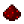# vectorA:dotFunction vector:dot Returns the dot product of two vectors. Syntax vector:dot(vector vect) Returns vector Part of ComputerCraft API vector

## ExamplesExample Creates a dot product of two vectors and prints the resulting vector. Code ```local a = vector.new(1, 2, 3) local b = vector.new(4, 5, 6) local c = a:dot(b) print(c) --32 ```Example Code to recreate the function. Gives insight into what the function does. Code ```local a = vector.new(1, 2, 3) local b = vector.new(-1, 2, 5) local c = ((a.x * b.x) + (a.y * b.y) + (a.z * b.z)) --c = 18 ```

In the case of dot products, a:dot(b) will equal b:dot(a).